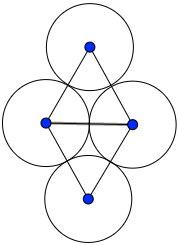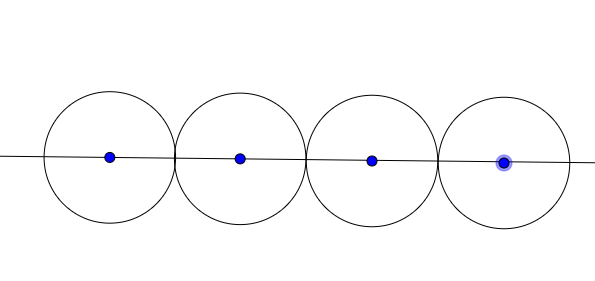# Four coins

Logic Level 3

There are four coins arranged as such:Note: The rhombus created by the centers of the circles is comprised of two equilateral triangles.

A move is defined to be moving a coin to another location such that at the end of the move, the coin that was moved is touching two other coins. Hence, how many moves are needed in order to obtain 4 coins in a straight line, like this? The orientation of the coins does not matter, the only condition is that their centers lie on one line.Enter -1 if it is impossible to do so. Bonus: Prove it!

×